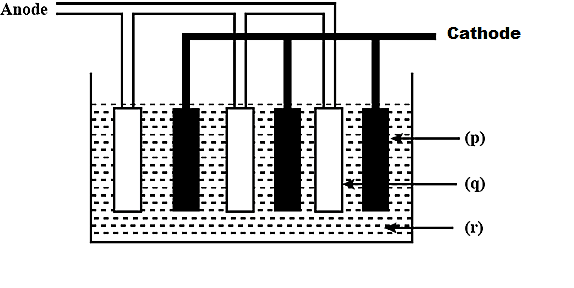# Label the given diagram showing lead storage battery.(A) ${\rm{p - Pb, q - Pb}}{{\rm{O}}_2},{\rm{r - 5M }}{{\rm{H}}_2}{\rm{S}}{{\rm{O}}_4}$(B) ${\rm{p - Pb}}{{\rm{O}}_{\rm{2}}}{\rm{,q - Pb,r - conc}}{\rm{.}}{{\rm{H}}_{\rm{2}}}{\rm{S}}{{\rm{O}}_{\rm{4}}}$(C) ${\rm{p - Pb, q - Pb}}{{\rm{O}}_2},{\rm{r - 50\% }}{{\rm{H}}_2}{\rm{S}}{{\rm{O}}_4}$(D) ${\rm{p - Pb}}{{\rm{O}}_{\rm{2}}}{\rm{,q - Pb,r - dil}}{\rm{. 38\% }}{{\rm{H}}_{\rm{2}}}{\rm{S}}{{\rm{O}}_{\rm{4}}}$Verified
198.9k+ views
Hint:. Lead storage batteries are also known as the lead acid battery. It is a kind of rechargeable battery. Lead storage batteries are the energy storage device. It is a secondary storage battery. This secondary storage battery can undergo reversible chemical reaction.

Complete step by step answer:
- Lead storage battery is also known as the secondary storage battery. In the lead storage battery, the electrical energy will be converted into chemical energy by the application of certain external sources. This is known as charging.
- Similarly, the chemical energy will be converted into electrical energy whenever it is required. This is called discharging.
- In the lead storage battery, both charging and discharging can take place.
- During the discharging process, both positive and the negative plate will be converted into ${\rm{PbS}}{{\rm{O}}_4}$. The electrolyte solution, i.e. dissolved ${{\rm{H}}_{\rm{2}}}{\rm{S}}{{\rm{O}}_{\rm{4}}}$will be lost and it will become water.
- The electron conduction can take place from the negative plate to the positive plate.
- The chemical reaction taking place during the discharging process is given below:
The reaction in the negative plate is
${\rm{Pb(s) + HSO}}_{\rm{4}}^{\rm{ - }}{\rm{(aq)}} \to {\rm{PbS}}{{\rm{O}}_{\rm{4}}}{\rm{(s) + }}{{\rm{H}}^{\rm{ + }}}{\rm{(aq) + 2}}{{\rm{e}}^{\rm{ - }}}$

The reaction in the positive plate is :
${\rm{Pb}}{{\rm{O}}_2}{\rm{(s) + HSO}}_{\rm{4}}^{\rm{ - }}{\rm{(aq) + 3}}{{\rm{H}}^{\rm{ + }}}{\rm{ + 2}}{{\rm{e}}^{\rm{ - }}} \to {\rm{PbS}}{{\rm{O}}_{\rm{4}}}{\rm{(s) + }}{{\rm{H}}_{\rm{2}}}{\rm{O(l)}}$
The overall reaction is :
${\rm{Pb(s) + Pb}}{{\rm{O}}_{\rm{2}}}{\rm{(s) + 2}}{{\rm{H}}^{\rm{ + }}}{\rm{ + 2HSO}}_{\rm{4}}^{\rm{ - }} \to {\rm{PbS}}{{\rm{O}}_{\rm{4}}}{\rm{(s) + }}{{\rm{H}}_{\rm{2}}}{\rm{O(l)}}$
The charging process is reverse of discharging process.
Hence,
${\rm{p - Pb}}{{\rm{O}}_{\rm{2}}}$,
${\rm{q - Pb}}$,
${\rm{r - dil}}{\rm{. 38\% }}{{\rm{H}}_{\rm{2}}}{\rm{S}}{{\rm{O}}_{\rm{4}}}$
Therefore, the correct answer is option (D) ${\rm{p - Pb}}{{\rm{O}}_{\rm{2}}}{\rm{,q - Pb,r - dil}}{\rm{. 38\% }}{{\rm{H}}_{\rm{2}}}{\rm{S}}{{\rm{O}}_{\rm{4}}}$

Additional information: Comparison between Primary cell and Secondary cell:
 PRIMARY CELL SECONDARY CELL It has high density and is very easy to use. It has a very low density Primary cell is not rechargeable. Secondary cell is rechargeable It is very Cheap It is costly. It has high internal resistance It has low internal resistance. It undergoes irreversible chemical reaction It undergoes reversible chemical reaction

Note: Lead storage batteries are composed of very harmful chemicals. If it is not maintained properly, it can be hazardous to our health. The sulphuric acid used in the battery is highly corrosive in nature. Therefore, after the end life of the battery, it has to be disposed properly.